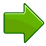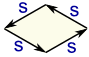# Rhombus

A Rhombus is a flat shape with 4 equal straight sides.A rhombus looks like a diamondAll sides have equal lengthOpposite sides are parallel, and opposite angles are equal (it is a Parallelogram).The altitude is the distance at right angles to two sidesAnd the diagonals "p" and "q" of a rhombus bisect each other at right angles.Play with a rhombus:

## Area of a RhombusThe Area can be calculated by:

• the altitude times the side length:
Area = altitude × s

• the side length squared (s2) times the sine of angle A (or angle B):
Area = s2 sin(A)
Area = s2 sin(B)

• by multiplying the lengths of the diagonals and then dividing by 2:
Area = (p × q)/2

### Example: A rhombus has diagonals of 6 m and 8 m, what is its Area?

Area = (6 m × 8 m)/2 = 24 m2

If you can draw your Rhombus, try the Area of Polygon by Drawing tool.

## Perimeter of a Rhombus

The Perimeter is the distance around the edges.The Perimeter is 4 times "s" (the side length) because all sides are equal in length: Perimeter = 4s

### Example: A rhombus has a side length of 12 cm, what is its Perimeter?

Perimeter = 4 × 12 cm = 48 cm

## Is a Square a Rhombus?

Yes, because a square is just a rhombus where the angles are all right angles.

## Other Names

It is more common to call this shape a rhombus, but some people call it a rhomb or even a diamond.

The plural is rhombi or rhombuses, and, rarely, rhombbi or rhombbuses (with a double b).

The name "rhombus" comes from the Greek word rhombos: a piece of wood whirled on a string to make a roaring noise!